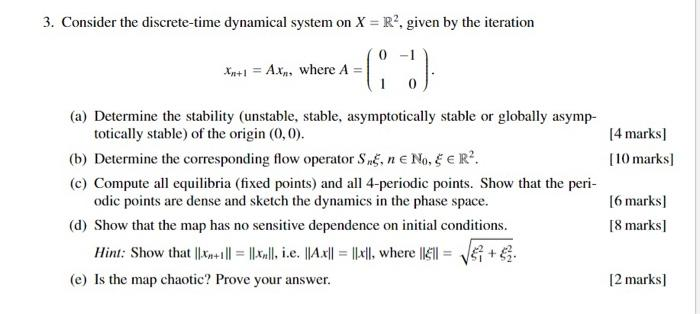# Question please help 3. Consider the discrete-time dynamical system on X = RP. given by the iteration Xn+1 = A.x,, where A -G :) [4 marks) [10 marks) (a) Determine the stability (unstable, stable, asymptotically stable or globally asymp- totically stable) of the origin (0,0). (b) Determine the corresponding flow operator Sn, ne NO, E ER? (c) Compute all equilibria (fixed points) and all 4-periodic points. Show that the peri- odic points are dense and sketch the dynamics in the phase space. (d) Show that the map has no sensitive dependence on initial conditions. Hint: Show that ||xn+1 || = ||xn||, i.e. || 4.4|| = ||«l], where 11611 = +€ (e) is the map chaotic? Prove your answer. 16 marks) [8 marks [2 marks